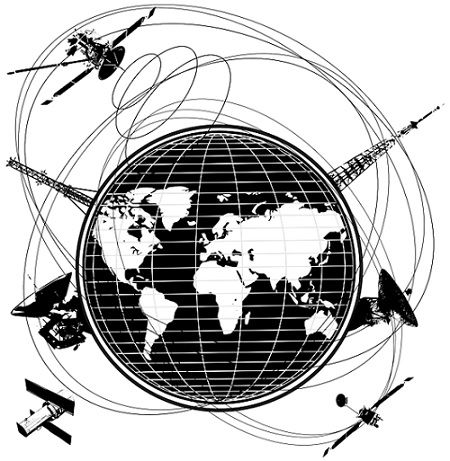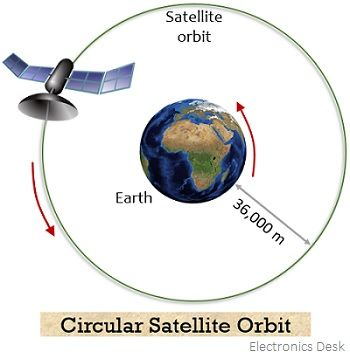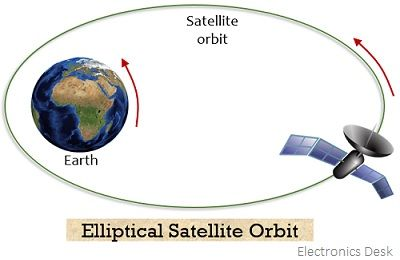# Satellite Orbits

Definition: The motion of satellites around the earth can be done through various paths, so, the paths in which the satellite is rotated around the earth are known as Satellite Orbits. These are also known as orbits of satellites. The various paths followed by the satellites have their own significance because of their respective applications.

The direction of rotation of satellite around the earth can be either same as the direction in which the earth is moving or opposite to the direction of rotation of the earth. So, the orbit that allows rotation of satellite in a similar direction to that of rotation of the earth is called prograde while the one in which direction of rotation of satellite is opposite to the direction of earth’s rotation is retrograde.

## Gravity and Satellite Orbits

We all are aware of the basic law of Gravitation proposed by Newton that anything that is thrown upwards comes back to the ground due to the gravitational pull of the earth. In a similar way when a satellite is vertically launched from the surface of earth then due to gravity it will also fall back to the ground.

So, to achieve orbital motion of the satellite around the earth there must be some forward motion. Due to this reason; the launch should be initiated in a straight manner in the forward direction. Basically, the forward motion results in inertia due to which the satellite moves in a straight line but the gravity of the earth tries to pull the satellite towards the surface of the earth. And these two effects in combination give rise to centripetal acceleration. More specifically, we can say, that the centripetal force is the result of the inertia of the satellite along with gravity that keeps the motion of the satellite in the desired orbit.

Also, when the launch speed is very high then the satellite can travel from earth to space. Now, the question arises, how at higher speed the satellite can reach space when launched from earth.

Basically, at an extremely high speed of around 42,075 km/hr, the gravitational pull of the earth vanishes which constantly exists at lower speeds. Thus, with such a high launch speed, the satellite can reach space.Another noteworthy point over here is that closer the orbit of the satellite more the gravitational pull it will experience. Thus, in the case of lower orbits, the satellite must possess high velocity.

### Fundamentals of Satellite Orbit

We have recently discussed that the orbital position of the satellite is maintained due to the balancing between the centripetal force of the satellite and the gravitational attraction of the earth. However, you must note here that there are four crucial factors on which this balancing depends which are as follows:

• The speed of a satellite in motion,
• The distance from the surface of the earth,
• The radius of the earth and,
• Earth’s gravitational force.

The satellite orbits are nothing but the path followed by satellite while revolving around the earth and these are generally classified into two categories:The radius of the satellite orbits is the distance between the center of the earth to the satellite in the present in the orbit. The altitude and inclination of the orbit denote the height of the satellite from the earth’s surface and the angle of rotation of the satellite with the horizon, respectively.

## Types of Satellite Orbits

The figure below represents the circular orbital shape of the satellite:Also, the elliptical satellite orbit is given as:As it is clear from the above figure that the radius of the elliptical orbit is variable but that of a circular orbit is constant. And the behavior of the satellites in the orbit follows Kepler’s law of planetary motion.

The circular orbit is further classified into 3 categories which are as follows:

Geostationary Orbit (GEO):  A geostationary orbit is generally present at an altitude of 35,786 km. When a satellite is present in geostationary orbit then to a distant earth-based observer it appears to be at a fixed position. This is so because a satellite in geostationary orbit revolves around earth at a constant speed generally with the earth’s angular velocity. Thus, the satellites orbiting in this orbit is known as geostationary. This circular orbit is present exactly at the equatorial plane of earth.

It is to be noted here that the earth takes 23 hours and 56 minutes to revolve around its axis. Thus, the geostationary satellite must possess rotation of the same period around earth i.e., 23 hours and 56 minutes.

These satellites suit uses mainly in the field of communication because the ground-based antennas easily support effective communication without needing costly equipment.Medium Earth Orbit (MEO): This earth orbit region lies below GEO but above LEO and possesses an altitude between 2000 to 36000 km. It is also given the name intermediate circular orbit. The most common altitude of this orbit is 20,200 km and possesses an orbital period of about 2 to 24 hours.

These are mostly used for the purpose of navigation, communication, and monitoring, etc. The satellites that operate in this orbit are generally Galileo, GPS, Telstar, etc.

Low Earth Orbit (LEO): This circular orbit exists at 400 to 900 km above the surface of the earth. This orbit exists in close proximity to the surface of the earth. Also, despite having an altitude less than 1000 km, it exists far away from the surface of the earth.

LEO is a very commonly used orbit due to the reason that as GEO exists on the earth’s equator thus satellites have to move in a specific path. But this is not the case with LEO as their plane can be titled. This provides more available routes for the movement of satellites in the orbit.

Satellites in LEO exhibit an orbital period of around 90 minutes and finds applications like satellite imaging. Basically, the close presence of the orbit from the surface of the earth allows the satellites to capture images of high resolution. But these are not suitable for telecommunication applications as they move with high speed around the earth and this makes their tracking quite difficult from stations present at the earth’s surface. Generally, satellite constellation is formed by LEO satellites.

### Station Keeping

Station keeping is basically a technique used to overcome orbital drift. Orbital drift may arise due to various factors. Like the sun and moon hinder the position of the satellite due to gravitational pull applied by them. Also, the earth possesses the shape of an oblate spheroid rather than a perfect sphere thus it offers inconsistent gravitational field at different points on the earth’s surface. This results in orbital drift and this requires periodic satellite orbit adjustment. This orbital adjustment is called station keeping.